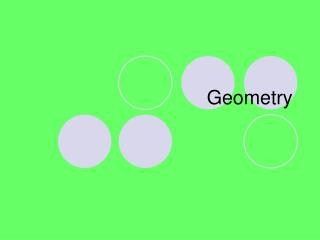DownloadDownload PresentationGeometry

# Geometry

Télécharger la présentation## Geometry

- - - - - - - - - - - - - - - - - - - - - - - - - - - E N D - - - - - - - - - - - - - - - - - - - - - - - - - - -
##### Presentation Transcript

1. Geometry

2. Line • Never ending • (NO NOTES) • ↔

3. Line Segment • Has two endpoints • (NO NOTES) • ●▬●

4. Point • A place on a line • (NO NOTES) • ●

5. Plane • An area with geometric figures • Also called a coordinate plane

6. Ray • Consist of an endpoint and a line • Used to create angles • →

7. Angle • Consist of two rays that meet at a vertex • (NO NOTES) • ∟

8. Vertex • The point that two rays have in common • Used to form angles and polygons

9. Acute • An angle less than 90 degrees • Used to classify angles and triangles

10. Right • An angle that measures exactly 90 degrees • Used to classify angles and triangles

11. Obtuse • An angle that measures greater than 90 degrees but less than 180 degrees • Used to classify angles and triangles

12. Triangle • Consist of three angles that when added equals 180 degrees • A= 45, B = 45, C = 90 A + B + C = 180 45 + 45 + 90 = 180 180 = 180

13. Quadrilateral • Is made of four segments that intersect only at their endpoints • Has four vertices that when added equals 360 degrees

14. Congruent Segments • Have equal lengths • Used to classify polygons

15. Congruent Angles • Have equal measures • Used to classify polygons

16. Straight Angle • An angle that measures exactly 180 degrees • A straight line

17. Reflex Angle • An angle that measures more than 180 degrees but less than 360 degrees • Pay attention to notation (angle symbol)

18. Complementary Angles • Two angles that add up to 90 degrees • Calculate by subtracting the given angle from 90

19. Supplementary Angles • Two angles that add up to 180 degrees • Calculate by subtracting the given angle from 180

20. Vertical Angles • Angles opposite each other when two straight lines cross • (NO NOTES)

21. Perpendicular Lines • When two lines meet or cross at a right angle • (NO NOTES)

22. Parallel Lines • Lines that run side by side and never cross • (NO NOTES)

23. Transversal Line • A third line that crosses two parallel lines • (NO NOTES)

24. Equilateral Triangle • Has three equal sides and three equal 60 degree angles • (NO NOTES)

25. Isosceles Triangle • Has two equal sides and two equal angles called base angles, the third angle is called the vertex angle • (NO NOTES)

26. Scalene Triangle • Has no equal sides and no equal angles • (NO NOTES)

27. Right Triangle • An isosceles or scalene triangle that has a 90 degree angle • (NO NOTES)

28. Similar Triangles • Have the same shape but not necessarily the same size • Differ only in the lengths of their sides • All angles are equal

29. Corresponding Sides • Sides or angles that have the same relative position • The sides that are opposite the equal angles • The corresponding sides can be written as a proportion

30. Square • Of a number, is that number multiplied by itself • 6 is the base and 2 is the exponent • 6 is called the factor • 6 x 6 = 36 • 62

31. Perfect Square • Numbers that have whole numbers as their square roots • (NO NOTES) • Example: 152 = 225

32. Square Roots • Symbol √ (radical) • Think: What number times itself equals this number? • Example √25 = 5

33. Hypotenuse • In a right triangle the side opposite the right angle • (NO NOTES)

34. Pythagorean Theorem • Relationship between the hypotenuse and the two shorter sides • Formula – c2 = a2 + b2

35. Circle • Figure with all points the same distance from the center • Equals 360 degrees

36. Radius • From the center of the circle to the side • Symbol – r • Equals ½ of the diameter

37. Diameter • Distance across the circle, from side to side going through the center • Symbol – d • Equals two times the radius

38. Circumference • The distance around the circle • Formulas • Radius – C = 2∏r • Diameter – C = ∏d

39. Pi • Greek letter • ∏ • Approximate value is 3.14 or 22/7

40. Area • Measure of surface, measured in square units • Formulas • Rectangle – A=lw • Square – A =s2 • Triangle – A = 1/2bh • Circle – A = ∏r2 • A = lw • A=6 * 10 • A = 60 squared units

41. Volume • Measure of the space taken by a solid object • measured in volume units (cubic) • most common shape is the rectangular solid • Formulas • Rectangular solid V= lwh • Cylinder V = ∏r2h • Example • l=4, h = 2, w= 1 • V=lwh • V=4*2*1 • V=8 cubic inches

42. Perimeter • Distance around a shape • Formula • Square -- P = 4s • Rectangle -- P = 2l + 2w • Triangle –- P = s + s + s • Example • P=4s • P=4*4 • P=16 inches